# Applied Mathematics

##### Prof. Nihat Ay (TUHH)Research interests and expertise
• Information geometry and its applications to biology
• Mathematical theory of learning in the context of neural networks, cognitive systems, and robotics
• Machine learning
• Graphical models (Bayesian networks) and their applications to causality theory
• Complexity and information theory
• Geometric structures in quantum information theory
Potential match-making fields
• Inverse problems (in particular, in the context of scattering and diffraction patterns)
• Neuronal representation and inference of physical structures and laws
• Causal inference
• Prediction and active inference
Publications

### Applied Analysis

##### Dr. Christian Seifert (TUHH)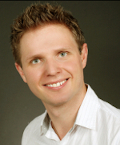Research interests and expertise
• Applied Functional Analysis
• Mathematical Physics
• Partial Differential Equations
• Evolution Equations
• Approximation
• Influence of Randomness
• Uncertainty Quantification
• Neural Networks
Potential match-making fields
• Data Science
• Machine Learning
Publications

### Computational Mathematics

##### Prof. Sabine Le Borne (TUHH)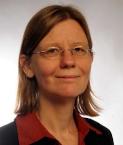Research interests and expertise
• numerical linear algebra
• iterative solvers
• preconditioners
• rank structured/hierarchical matrices
• data fitting
Potential match-making fields
• modeling and simulation with partial differential equations
• population balance equations
• multivariate aggregation
Publications
##### Prof. Daniel Ruprecht (TUHH)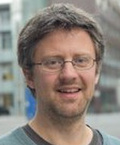Research interests and expertise
• omputational mathematics and numerical algorithms
• high-performance computing
• parallel-in-time integration methods
• time-dependent differential equations and initial value problems
• optimisation
Potential match-making fields
• modeling and simulation with partial differential equations
• modelling and simulation with time-dependent differential equations
• simulations of fast ion trajectories
Publications

### Discrete Mathematics

##### Prof. Anusch Taraz (TUHH)Research interests and expertise
• Discrete Mathematics
• Combinatorial Optimization
• Random Discrete Structures
• Randomized Algorithms
• Probabilistic and Extremal Combinatorics
Potential match-making fields
• Complex Networks
• Random Graphs
• Average-case Analysis of Algorithms
• Positional Games
Publications

### Intelligent Process Control

##### Dr. Annika Eichler (DESY)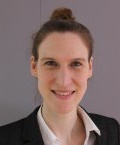Research interests and expertise
• control theory
• feedback controller design for stability and robustness
• fault diagnosis
• supervisory control
• mode predictive control
• merging data-driven with model-based control and diagnosis techniques
• reinforcement leaning and dynamic programming
• distributed control concepts and distributed optimization
Potential match-making fields
• machine learning
• data sciences
• predictive maintenance
• optimization
• computer science
Publications

### Numerical Mathematics

##### Prof. Michael Breuer (HSU)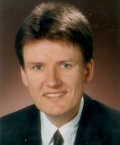Research interests and expertise
• fluid mechanics
• turbulence
• fluid-structure interaction
• multiphase flows
• computational fluid dynamics
• large-eddy simulation
• direct numerical simulation
• high-performance computing
Potential match-making fields
• modeling and simulation with partial differential equations
• numerical algorithms for continuum mechanics and particle tracking
• multiphysics
• high-performance computing
Publications
##### Prof. Armin Iske (UHH)Research interests and expertise
• Approximation theory and algorithms for mathematical data analysis
• Kernel-based multivariate scattered data approximation
• Meshfree methods for partial differential equations
• Geometrical methods in dimensionality reduction
Potential match-making fields
• Applications of particle methods in fluid flow simulation
• Mathematical signal processing
• Adaptive approximation for sparse data representations
Publications

##### Dr. Jens-Peter Zemke (TUHH)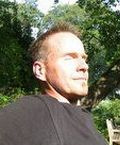Research interests and expertise
• numerical linear algebra
• Krylov subspace methods
• eigenvalue problems
• perturbation theory
• round-off error analysis
Potential match-making fields
• structure and symmetries
• initialization and training of neural networks
• fast implementation of numerical schemes
• optimization and regularization
Publications

### Optimization and Approximation

##### Prof. Christina Brandt (UHH)
Research interests and expertise
• inverse problems
• regularization methods (in particular variational regularization)
• convex optimization
• tomographic applications
• imaging
• magnetic particle imaging
Potential match-making fields
• Inverse problems: denoising, deconvolution problems, phase retrieval etc.
• Parameter identification
Publications

### Probability Theory and Mathematical Statistics

##### Prof. Mathias Trabs (formerly UHH)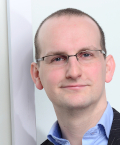Research interests and expertise
• mathematical statistics,
• especially high-dimensional and non-parametric statistics and statistics for stochastic processes
• stochastic processes,
• especially stochastic (partial) differential equations and Lévy processes
Potential match-making fields
• applications of stochastic processes and machine learning
Publications